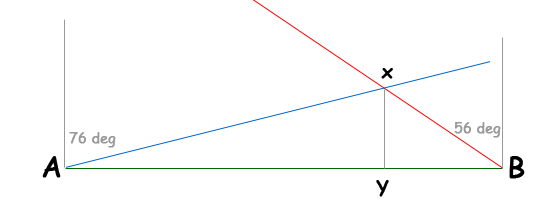SEARCH HOMEMath Central Quandaries & QueriesQuestion from tony, a student: Two fire towers are 30km apart, tower A is due west of tower B. A fire is spotted from the towers, and the bearing from A and B are N76degreesE and N56degreesW, respectively. Find the distance from the fire to the straight line connecting tower A to tower B.Hi Tony. Perhaps this diagram will help. North is upwards.You want the length of segment XY.

First find the interior angle measurements of triangle ABX. Then you can use the Law of Sines to calculate the length of segment BX. Once you have that, the last step is to use it in the right triangle BXY in a trig function of angle YBX to calculate the length XY.

Hope this helps,
Stephen La Rocque.Math Central is supported by the University of Regina and The Pacific Institute for the Mathematical Sciences.